# RS Aggarwal Solutions for Class 6 Maths Chapter 16 Triangles Exercise 16A

Exercise 16A of Chapter 16 explains fundamental concepts like types of triangle, some important results and angle sum property of a triangle. We know that the triangle is a figure which has three sides and three angles. The various types of triangles which are explained in RS Aggarwal Solutions help students gain an overall idea about the concepts. The students can self analyse their performance using RS Aggarwal Solutions for Class 6 Maths, Chapter 16 Triangles Exercise 16A PDF, which is available here.

## Download PDF of RS Aggarwal Solutions for Class 6 Maths Chapter 16 Triangles Exercise 16A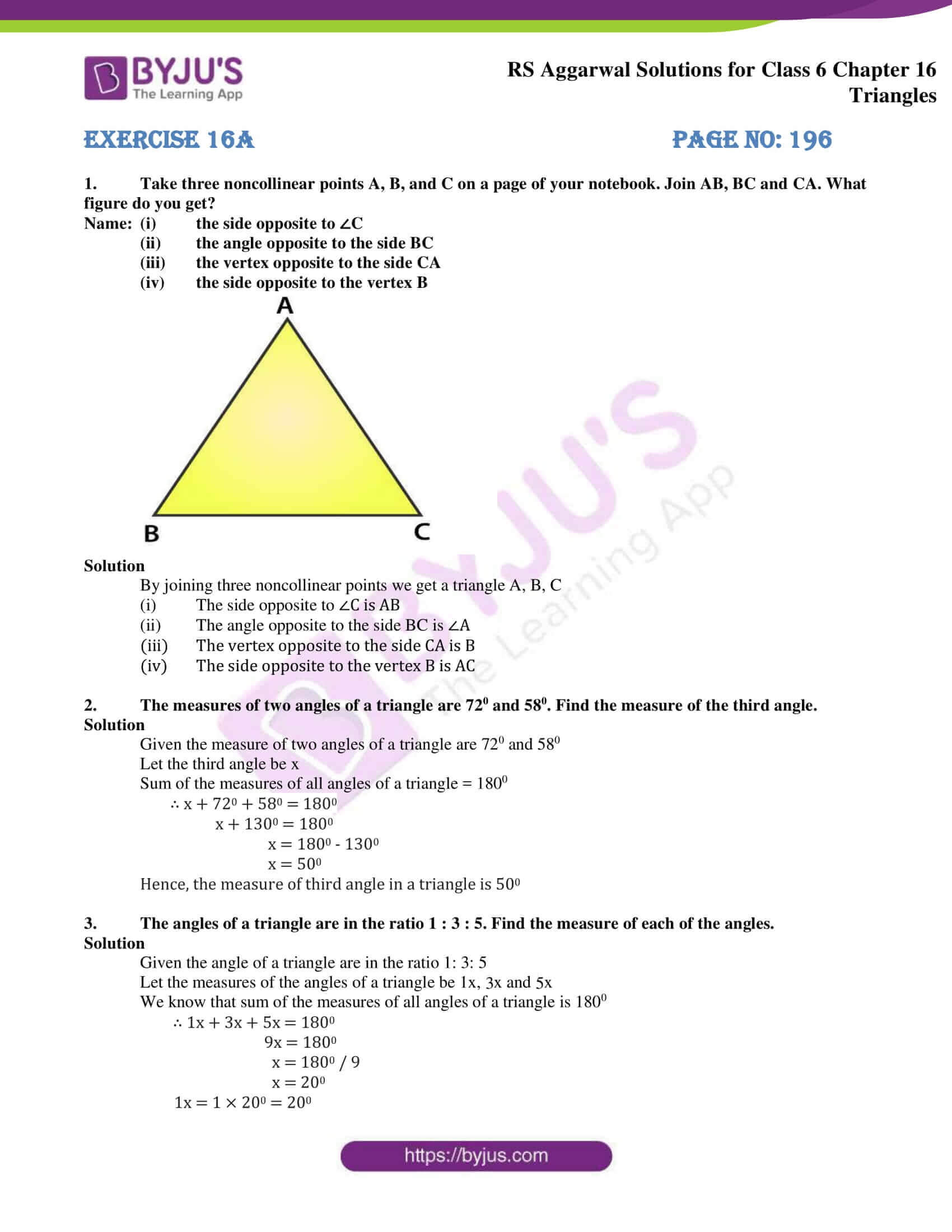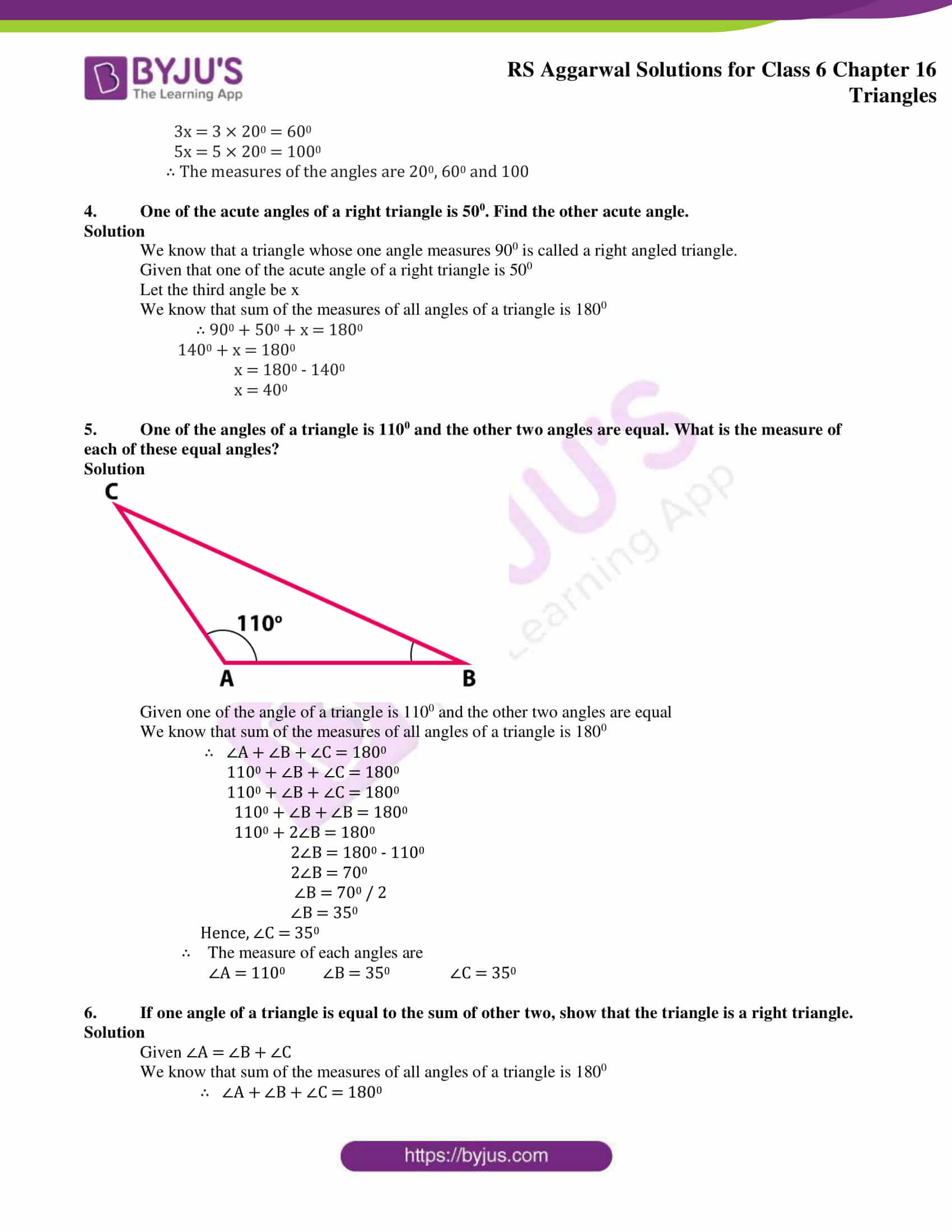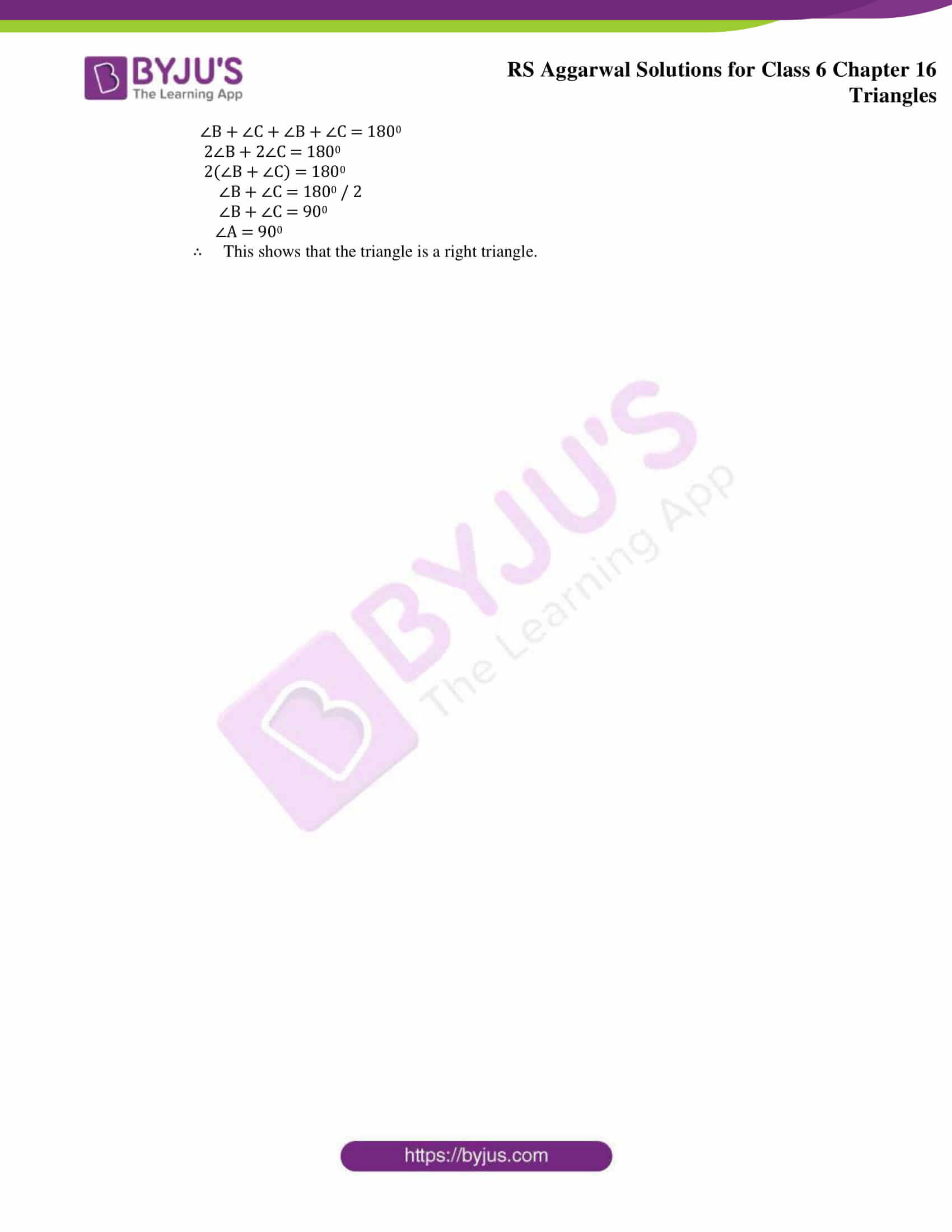### Access answers to Maths RS Aggarwal Solutions for Class 6 Chapter 16 Triangles Exercise 16A

1. Take three noncollinear points A, B, and C on a page of your notebook. Join AB, BC and CA. What figure do you get?

Name: (i) the side opposite to ∠C

(ii) the angle opposite to the side BC

(iii) the vertex opposite to the side CA

(iv) the side opposite to the vertex B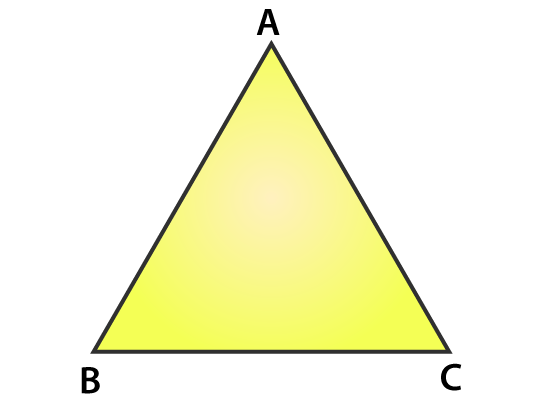Solution

By joining three noncollinear points we get a triangle A, B, C

(i) The side opposite to ∠C is AB

(ii) The angle opposite to the side BC is ∠A

(iii) The vertex opposite to the side CA is B

(iv) The side opposite to the vertex B is AC

2. The measures of two angles of a triangle are 720 and 580. Find the measure of the third angle.

Solution

Given the measure of two angles of a triangle are 720 and 580

Let the third angle be x

Sum of the measures of all angles of a triangle = 1800

∴ x + 720 + 580 = 1800

x + 1300 = 1800

x = 1800 – 1300

x = 500

Hence, the measure of third angle in a triangle is 500

3. The angles of a triangle are in the ratio 1 : 3 : 5. Find the measure of each of the angles.

Solution

Given the angle of a triangle are in the ratio 1: 3: 5

Let the measures of the angles of a triangle be 1x, 3x and 5x

We know that sum of the measures of all angles of a triangle is 1800

∴ 1x + 3x + 5x = 1800

9x = 1800

x = 1800 / 9

x = 200

1x = 1 × 200 = 200

3x = 3 × 200 = 600

5x = 5 × 200 = 1000

∴ The measures of the angles are 200, 600 and 100

4. One of the acute angles of a right triangle is 500. Find the other acute angle.

Solution

We know that a triangle whose one angle measures 900 is called a right angled triangle.

Given that one of the acute angle of a right triangle is 500

Let the third angle be x

We know that sum of the measures of all angles of a triangle is 1800

∴ 900 + 500 + x = 1800

1400 + x = 1800

x = 1800 – 1400

x = 400

5. One of the angles of a triangle is 1100 and the other two angles are equal. What is the measure of each of these equal angles?

Solution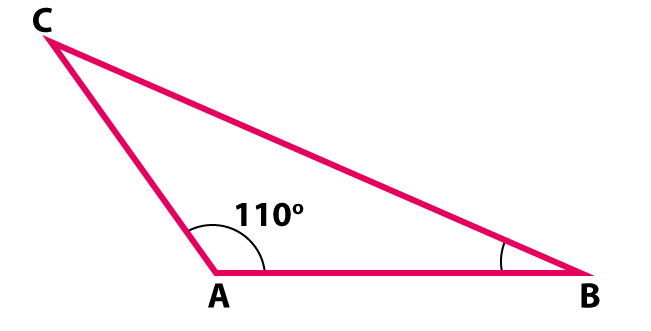Given one of the angle of a triangle is 1100 and the other two angles are equal

We know that sum of the measures of all angles of a triangle is 1800

∴ ∠A + ∠B + ∠C = 1800

1100 + ∠B + ∠C = 1800

1100 + ∠B + ∠C = 1800

1100 + ∠B + ∠B = 1800

1100 + 2∠B = 1800

2∠B = 1800 – 1100

2∠B = 700

∠B = 700 / 2

∠B = 350

Hence, ∠C = 350

∴ The measure of each angles are

∠A = 1100 ∠B = 350 ∠C = 350

6. If one angle of a triangle is equal to the sum of other two, show that the triangle is a right triangle.

Solution

Given ∠A = ∠B + ∠C

We know that sum of the measures of all angles of a triangle is 1800

∴ ∠A + ∠B + ∠C = 1800

∠B + ∠C + ∠B + ∠C = 1800

2∠B + 2∠C = 1800

2(∠B + ∠C) = 1800

∠B + ∠C = 1800 / 2

∠B + ∠C = 900

∠A = 900

∴ This shows that the triangle is a right triangle.

### Access other exercises of RS Aggarwal Solutions for Class 6 Maths Chapter 16 Triangles

Exercise 16B Solutions